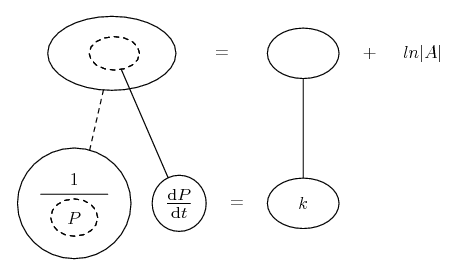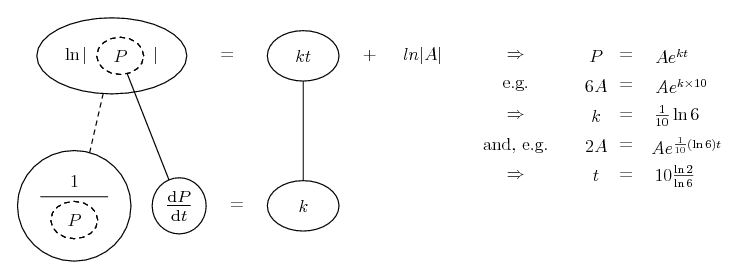# Thread: DE PRoblem

1. ## DE PRoblem

In a certain culture of bacteria, the number of bacteria increased sixfold in 10 h. How long did it take for the population to double?

I'm not even sure how this relates to differential equations... Any advice? Thanks.

2. Hmm... nice one!

I'll give it a try, although you should look for a second opinion, ok?

With $\displaystyle x_0$ denote the number of bacteria in the begining, $\displaystyle t=0.$

Rate of increase in bacteria population clearly depends on the number of bacteria: greater the number of bacteria, greater the increase in their number. This statement has nothing to do with mathematics, its pure common sense, but it is crucial information to have if one wants to solve the problem. Instead of increase lets use the term rate of growth (of the whole culture), representing the change in the number of bacteria in time, and denote it with $\displaystyle \frac{dx}{dt}.$

Then $\displaystyle x=x(t)$ denoting the number of bacteria in a certain moment in time can be calculated from the equation: $\displaystyle x(t)=x_0+t\cdot \frac{dx}{dx}.$ Number to begin with plus rate of growth in time multiplied by time elapsed from the beginning.

Now put to use the information given to you:
Sentence "the number of bacteria increased sixfold in 10 h" translates to the following equation: $\displaystyle 6x_0=x_0+10\cdot \frac{dx}{dt}$. This equation enables us to come up with the rate of growth: $\displaystyle \frac{dx}{dt}=\frac{x_0}{2}$. I mentioned that the rate of growth of bacteria depends on their number and this is the proof of that.

Now we have the formula for calculating the number of bacteria: $\displaystyle x(t)=x_0+t\cdot \frac{x_0}{2}$. Time needed for the number of bacteria to double can be translated to equation: find the value of variable $\displaystyle t$ such that $\displaystyle x(t)=2x_0.$

So, you have: $\displaystyle 2x_0=x_0+t\cdot \frac{x_2}{2}$. Divide by $\displaystyle x_0$ (you can do that since $\displaystyle x_0\neq 0$ because you had to have some bacteria to begin with in the first place). Now it follows:
$\displaystyle 2=1+t\cdot\frac{1}{2} \quad \Longrightarrow \quad t=2,$ so it would take 2 hours for the culture to double its numbers.

errr...I guess.

3. MathoMan, you reason correctly that the growth rate depends on P, but then you seem to make the population a linear function of t. Better to make growth rate a linear function of P, then do separation of variables. Then you'll arrive at the exponential growth formula,

$\displaystyle P = Ae^{kt}$

... where P is population, A the initial population, t the time elapsed and k the so-called 'growth constant'.

Then you can say,

$\displaystyle 6A = Ae^{k \times 10}$

and go from there to solve for k, and then solve...

$\displaystyle 2A = Ae^{kt}$

... for t.

The calculus to get the growth formula: start with your equation giving growth as a linear function of population,

$\displaystyle \displaystyle{\frac{\mathrm{d}P}{\mathrm{d}t} = kP}$

(No constant term, because: no population, no growth!)

Divide through by P, then you can integrate both sides with respect to t, using the chain rule on the left.

Just in case a picture helps...... where (key in spoiler) ...

Spoiler:... is the chain rule. Straight continuous lines differentiate downwards (integrate up) with respect to the main variable (in this case ), and the straight dashed line similarly but with respect to the dashed balloon expression (the inner function of the composite which is subject to the chain rule).

To settle ?(P) in the top balloon we need to integrate / anti-differentiate up the dashed line, i.e. with respect to the dashed balloon i.e. with respect to P. What function of P is it whose derivative is 1/P ? And on the right hand side, what function of t is it whose derivative (differentiating with respect to t) is a constant (i.e. k) ?

The plus ln|A| there on the right is the constant of integration. Calling it 'ln|A|' instead of the usual 'A' or 'C' will possibly help (a bit) when, after raising both sides of the top row as a power of e, you then try to simplify the right-hand side, to arrive at the exponential growth formula...

Spoiler:________________________________________

Don't integrate - balloontegrate!

Balloon Calculus; standard integrals, derivatives and methods

Balloon Calculus Drawing with LaTeX and Asymptote!

4. Tom, You're absolutely right! Everyone, discard my previous post.

#### Search Tags

problem